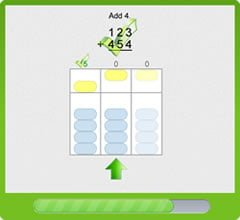# Numbers

## Numbers

Numbers instills a deep, intuitive sense for numbers. So much so that our goal is to teach every student how to easily add and subtract large numbers without using a pencil!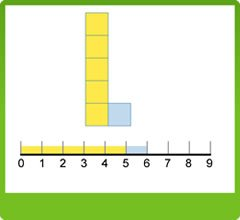## Six Modules

Numbers has six modules. Numbers Part 1 uses number manipulatives to give the student an intuitive understanding of composing and decomposing numbers. Numbers Part 2 introduces place value. Easy Add & Subtract uses the abacus to teach not only numbers but also adding and subtracting. By the time a student finishes the fifth module and completely masters the abacus, the student will have a deep intuitive sense of numbers.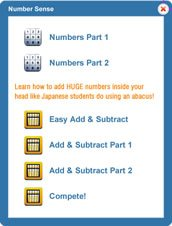## Track Student Progress

You can track student progress on both the individual student and class levels. You can see the amount of time a student works each day as well as their progress through each of the levels in each module.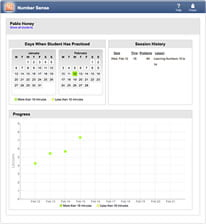## Touch Manipulatives

Each of the manipulatives are designed to not only see how numbers are composed and decomposed but actually get the "feel" of the number by moving each of the number's components. Though the touch manipulatives are a form of direct instruction, students become so engaged that teachers "have to pry their fingers off their tablets!"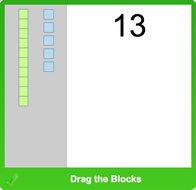## Abacus: The Ultimate Numbers

The abacus teaches students how to deftly compose and decompose numbers while having fun. While students compete to reach the highest level (the Fighting Ninja Panda!), they also learn how to add and subtract huge numbers in their heads!